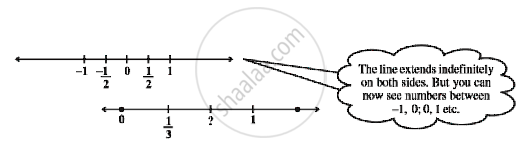Share

# Representation of Rational Numbers on the Number Line

#### notes

To represent rational number on a number line. Let us seeThe point on the first number line which is half way between 0 and 1 has been labelled 1/2 .
Also, the first of the equally spaced points that divides the distance between 0 and 1 into three equal parts can be labelled 1/3 , as on second  number line .
The point to be labelled is twice as far from and to the right of 0 as the point  labelled 1/3. So it is two times 1/3 , i.e. 2/3 .You can continue to label equally-spaced points on the number line in the same way. In this continuation, the next marking is 1. You can  see that 1 is the same as 3/3 .

### Shaalaa.com

Representation of Rational Numbers on the Number Line [00:08:26]
S
0%

S